# Linear Equations In Two Variables

## Pair Of Linear Equations In Two Variables of Class 10

1. The equation of the form ax = b or ax + b = 0, where a and b are two real numbers such that x ≠ 0 and x is a variable is called a inear equation in one variable.

2. The general form of a linear equation in two variables is ax + by +c = 0 or ax + by = c where a, b, c are real numbers and a ≠ 0, b ≠ 0 and x, y are variables.

3. The graph of a linear equation in two variables is a straight line.

4. The graph of a linear equation in one variable is a straight line parallel to x-axis for ay = b and parallel to y-axis for ax = b, where a ≠ 0.

5. A pair of linear equations in two variables is said to form a system of simultaneous linear equations.

6. The value of the variable x and y satisfying each one of the equations in a given system of linear equations in x and y simultaneously is called a solution of the system.

## Standard form of linear equation:

(Standard form refers to all positive coefficient)

a1x + b1y + c1 = 0 ....(i)

a2x + b2y + c2 = 0 ....(ii)

For solving such equations we have three methods.

(i) Elimination by substitution

(ii) Elimination by equating the coefficients

(iii) Elimination by cross multiplication.

### ELIMINATION BY SUBSTITUTION:

(Find the value of any one variable in terms of other and then use it to find other variable from the second equation):question 1. Solve x + 4y = 14 .....(i)

7x - 3y = 5 ....(ii)

Solution: From equation (i) x = 14 - 4y ....(iii)

Substitute the value of x in equation (ii)

⇒ 7 (14 - 4y) - 3y = 5

⇒ 98 - 28y - 3y = 5

⇒ 98 - 31y = 5

⇒ 93 = 31y

⇒ y = 93/31

⇒ y = 3

Now substitute value of y in equation (iii)

⇒ 7x - 3 (3) = 5

⇒ 7x - 3 (3) = 5

⇒ 7x = 14

⇒ x = 14/7 = 2

So the solution is x = 2 and y = 3

### ELIMINATION BY EQUATING THE COEFFICIENTS:

(Eliminating One variable by making the coefficient equal to get the value of one variable and then put it in any equation to find other variable):question 1. Solve 9x - 4y = 8 ...(i)

13x + 7y = 101 ...(ii)

Solution: Multiply equation (i) by 7 and equation (ii) by 4, we get

adding 63x – 28y = 56

52x + 28y = 404

115x = 460

⇒ .x = 460/115

⇒ x = 4

Substitute x = 4 in equation (i)

9 (4) –0 4y = 8 ⇒ 36 – 8 = 4y ⇒ 28 = 4y

⇒ y = 28/4 = 7

So the solution is x = 4 and y = 7.

### COMPARISON METHOD:

(Find the value of one variable from both the equation and equate them to get the value of other variable):

Let any pair of linear equations in two variables is of the form

a1x + b1y + c1 = 0 .........(i)

a2x + b2y + c2 = 0 .....…(ii)question 1. Solve for x and y : 2x + 3y = 8 , x - 5y = -9.

Solution: 2x + 3y = 8 …(i)

x - 5y = -9. …(ii)

From equation (i),

2x = 8 - 3y , x  = 8 - 3y/2  …(iii)

From equation (ii),

x = -9 + 5y…(iv)

From equation (iii) and (iv), we have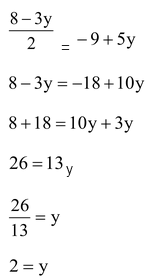Putting value of y in equation (iv), we have

x = 8 - 3 x 2/2

x = 1

Solution x = 1, y = 2.

### CROSS MULTIPLICATION METHOD:

Let the equation

a1x + b1y + c1 = 0 …(i)

a2x + b2y + c2 = 0 …(ii)

To obtain the values of x and y, we follow these steps:

1. Multiply Equation (i) by b2 and (ii) by b1, we get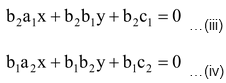2. Subtracting Equation (iv) from (iii), we get: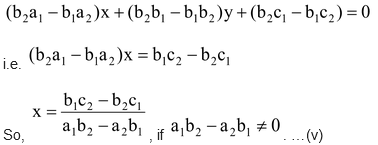3. Substituting this value of x in (i) or (ii), we get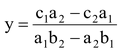. …(vi)

We can write the solution given by equations (v) and (vi) in the following form: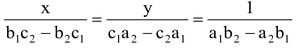…(vii)

In remembering the above result, the following diagram may be helpful: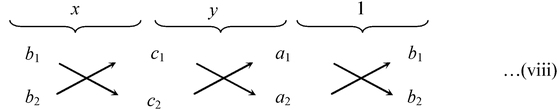For solving a pair of linear equations by this method, we will follow the following steps:

1. Write the given equations in the form (i) and (ii).

2. Taking the help of the diagram above, write equations as given in (viii).

3. Find x and y.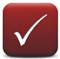For the equations like  a1x + b1y + c1 = 0 and a2x + b2y + c2 = 0 The solution set can be calculated by using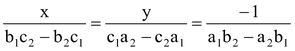(Please note position of c1 and c2 with equality sign and subsequent change in the third term i.e. –1)

question 1. Solve the following system of equations in x and y

ax + by - a + b = 0, bx - ay - a - b = 0

Solution: a1 = a, b1 = b, a2 = b and b2 = –a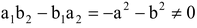Hence, the given system of the equation has unique solution.

By cross multiplication, we have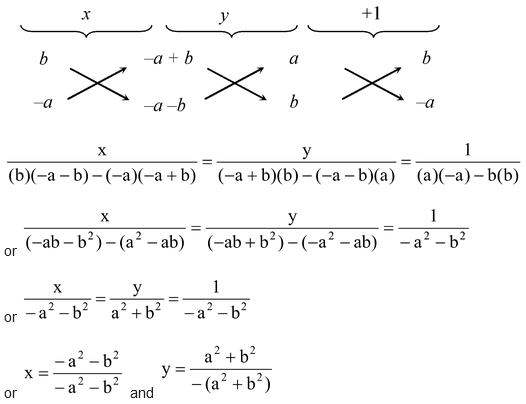or x = 1 and y = –1

question 2. Solve for x and y.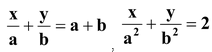Solution: Here a1 =1/a, b1 =1/b, a2 = 1/a2 and b2 = 1/b2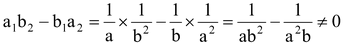.

Hence, the given system of equation has unique solution.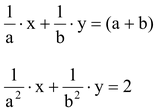By cross multiplication, we have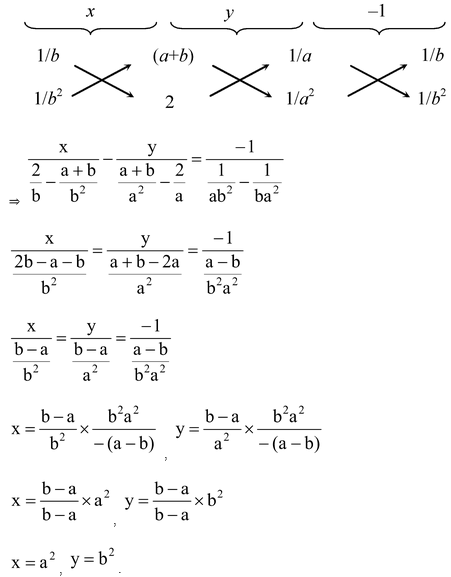question 3. Solve the following system of equations by using cross multiplication method:

5x + 3y = 19, 16x - 7y = 11

Solution: Here a1 = 5, b1 = 3 and a2 = 16, b2 = –7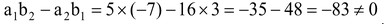.

Hence, the given system of equation has a unique solution. By cross multiplication, we have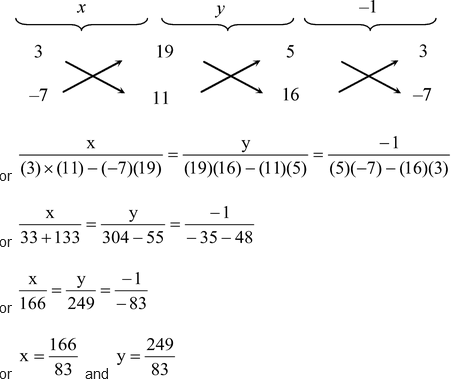or x = 2 and y = 3

Do solve NCERT text book for class 10 maths with the help of pw NCERT solutions for class 10 maths.